# Custom Table Calculations (Functions): Overview May 20, 2020 18:46

Follow

Custom table calculations (also called "Functions") make it easy to create on-the-fly metrics. They are similar to formulas found in spreadsheet tools like Excel. Custom table calculations appear as green columns in the data table, rather than as blue columns (dimensions), or orange columns (measures).

Custom table calculations can perform mathematical, logical (true/false), lexical (text-based), and date-based calculations on the dimensions, measures, and other custom table calculations in your query.

Notes:

• Custom Calculations only go up to 5000 rows. As a consequence, the Schedule and Send options will not have the option to pick All Results. Only Results in Table will be available under “Advanced options”, meaning that only the information available in the UI will be exported.
• To share reports in excess of 5000 rows, see our default sharing options in the articles Downloading and Sending Reports and Creating Schedules.

## Differences from Regular Fields

Although custom table calculations are similar to dimensions and measures, there are some important differences:

• Custom table calculations give anyone the ability to create new fields, as opposed to regular fields.
• Custom table calculations operate on the results from your query, as opposed to regular fields, which are part of the query itself. In other words, you’ll select a set of dimensions and measures and run your report as normal, then you can base custom table calculations on the data in that report.
• Since they can be created by anyone within your organization, they might not be the “official” calculations.

## Using Custom Table Calculations

On the custom reports, on your canvas, the dark Data bar has a Calculations button: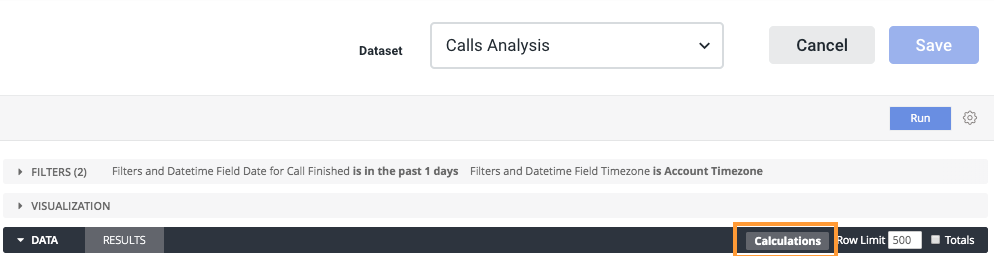In the custom table calculations pop-up window, you can start constructing your custom metrics. The expression you create can evaluate to a number, date, string (text), or boolean (true/false).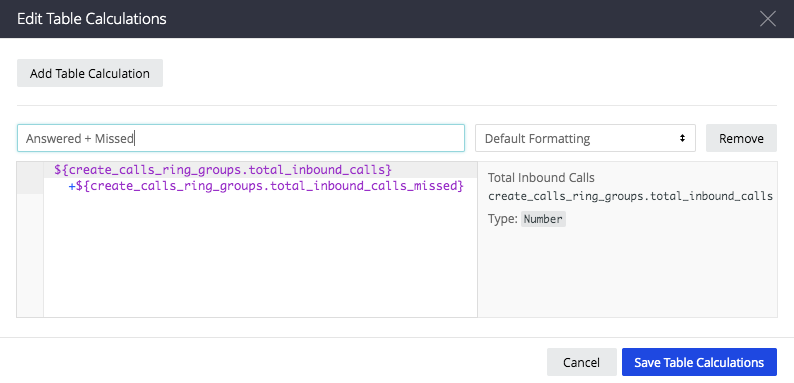If you already have some custom table calculations defined, click the Add custom table calculation button to create another. You are able to add as many custom table calculations as you need.

Then, for each custom table calculation:

1. Rename your custom table calculation if desired.
2. Optionally, click Default Formatting to choose a predefined format or create a custom format for the results. If you create a custom format, use Excel-style formatting as described on the article Custom Table Calculations, Formatting.
3. Start typing an expression into the large text box to form your calculation. Expressions can be quite simple, or they can use as many fields, functions and operators as your business logic requires. The article Custom Table Calculations, Creating Expressions
4.  explains how to create expressions and how the editor helps you.
5. If you are finished adding custom table calculations, click Save custom table calculations.

Your custom table calculation fields appear next to your dimensions and measures in the table. If you want to reuse your custom table calculations in the future, be sure to save your report or copy the custom table calculation formula into another document.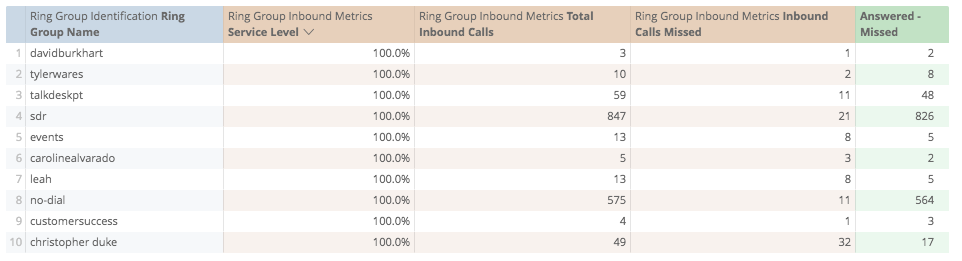## Sorting Custom Table Calculations

To sort on a custom table calculation, click the field name at the top of the column, just as you would a dimension or measure.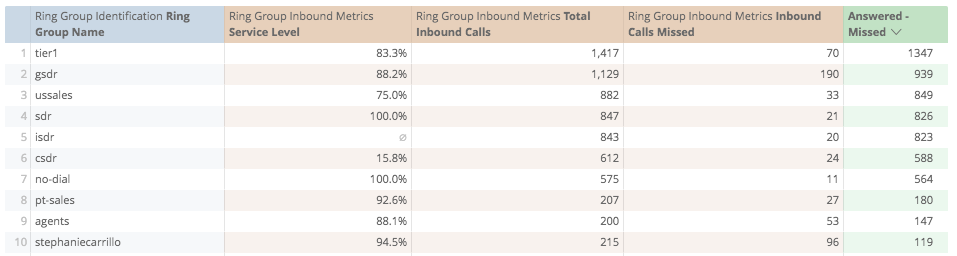## Limitations

Sorting on a custom table calculation works similarly to sorting on a dimension or measure through the Create feature. However, there are two important differences that prevent sorting in some scenarios:

• Calculations are based on query results. Custom table calculations are created after the data is retrieved from your database, which means that when you sort a custom table calculation, you can only sort the data that is already displayed.
• Sorting is disabled for order-dependent calculations. Some custom table calculations are applied to multiple rows within the same column (for example, when using an offset()function). In these cases, sorting the custom table calculation would change its results, and is therefore disabled.

The specific scenarios when you cannot  sort a custom table calculation are explained below:

### Calculations that Hit a Row Limit

If the number of rows in your query exceeds the row limit that you’ve set, you will not be able to sort custom table calculations. This is because custom table calculations are only based on the rows that are displayed. Therefore, if you hit a row limit, the custom table calculation might be missing some rows that it should be sorting into your results. If you run into this issue, you can try increasing your row limit (up to 5000 rows).

For example, the table below displays the top 10 Ring Groups and corresponding Service Level, sorted by the custom column field. Notice that the 10-row limit has been reached, which you’re warned about by the yellow bar displayed at the top of the table: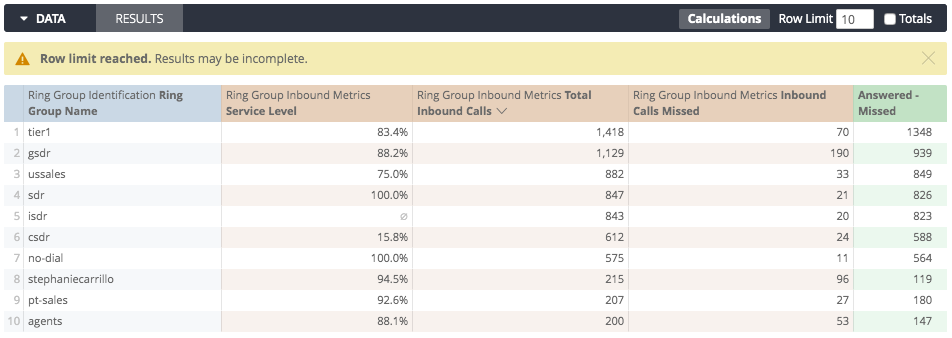However, if we want to show the top ten ring groups by number of total inbound calls instead, you can see the order of results change:If you had tried to use custom table calculations to do this, the calculations wouldn’t have searched through the undisplayed data, and would not have not re-ordered the distribution

### Sorting a Dimension or Measure after Sorting a Custom Table Calculation

As indicated above, custom table calculations are only based on the rows that are displayed. In contrast, sorting by a dimension measure goes back to your database to make sure it finds the correct rows. As a result, you should start sorting with dimensions and measures. Then, when the correct data has been returned from your database, you can sort those results based on a custom table calculation.

### Calculations Using an Offset Function cannot be Sorted

Any custom table calculation that makes use of an offset cannot be sorted, because the sort order of the rows would change the results of the offset.

For example, below is a custom table calculation that displays the change in weekly volumes for all the ring groups. And this only makes sense if the results are sorted by the week.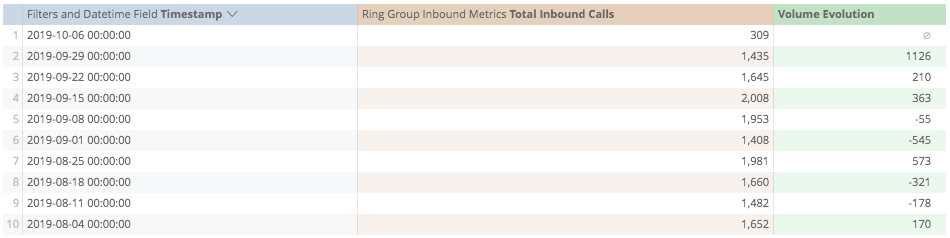## Using Custom Table Calculations in Visualizations

Just like regular dimensions and measures, custom table calculations are automatically displayed in visualizations.

In addition, you can use custom table calculations to decide which rows of your data should be displayed in a visualization. The example we’ll use to explore this feature is shown below and includes weekly volumes information for all ring groups. Note that the underlying data table includes the dimension Timestamp that returns the week, the measure Total Inbound Calls, and a custom table calculation called Volume Evolution that compares the volume of each week against the previous week:

##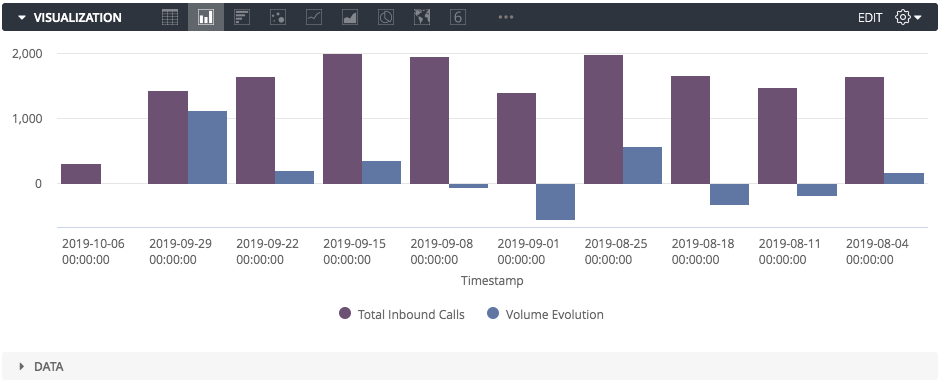We can now hide certain rows of data from showing up in the column chart. To do so, you’ll create a custom table calculation that evaluates to true or false, then hide the false values (which will appear as “no’s” in your data table). You don’t want the formula to result in the word “true” or “false”, rather it should be a condition that is either true or false.

For example, suppose we only want to show weeks that had greater volume than the previous week. We could create a custom table calculation called “Percent of Previous Week Volume” like this: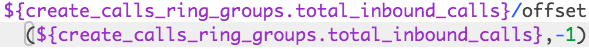Then we could create a custom table calculation called Exceeds Previous Week Volume like this: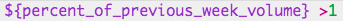This will result in a table that looks like this: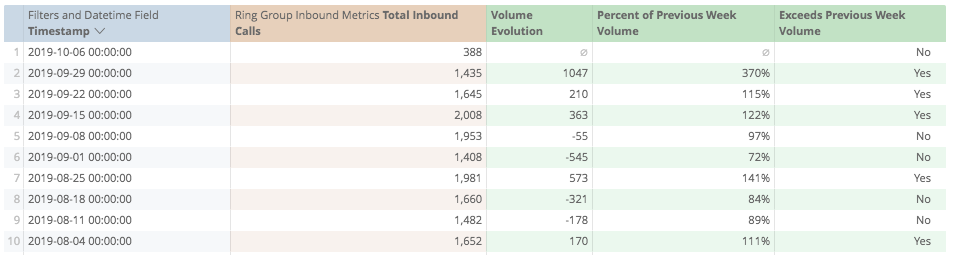To hide all of the rows where a particular week’s volume did not exceed the volume of the previous week, click the gear icon at the top left of the logical calculation and select Hide “No”s from Visualization: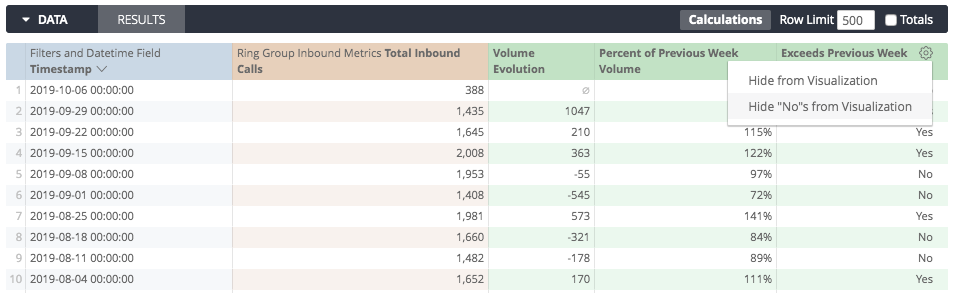The resulting visualization will now display only the weeks that exceeded the previous week’s volume: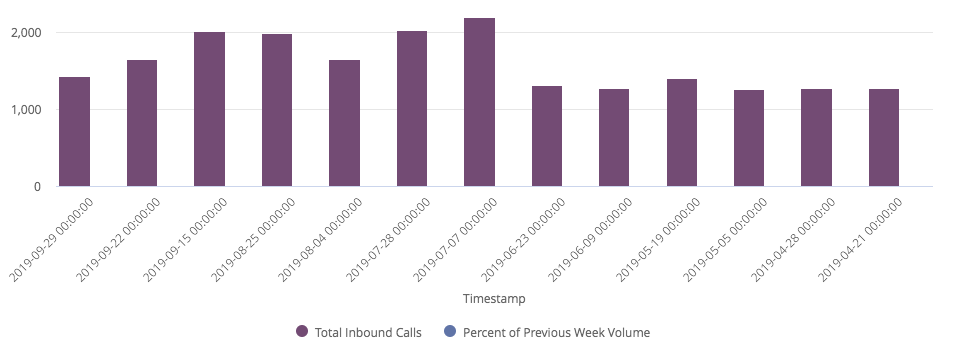One common use case for this feature is hiding the first or last row from a visualization, since many types of analysis create bad rows at the beginning or end of a table. For example, when you are calculating running totals, have a partial day ending a date analysis, or are calculating a percent of the previous row, like this example: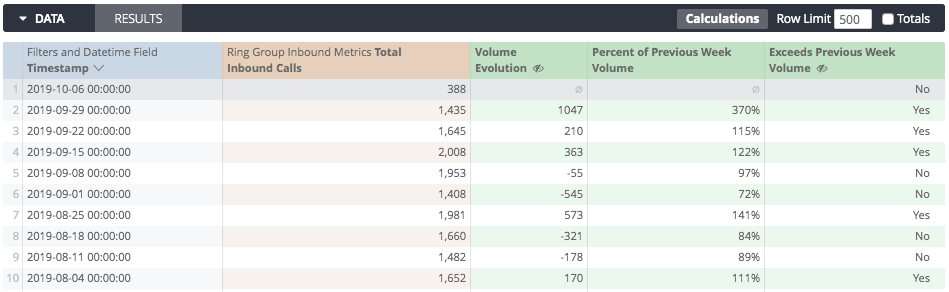To get rid of that row, simply create a new custom table calculation to filter out the null value: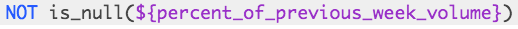Then, hide the row as normal: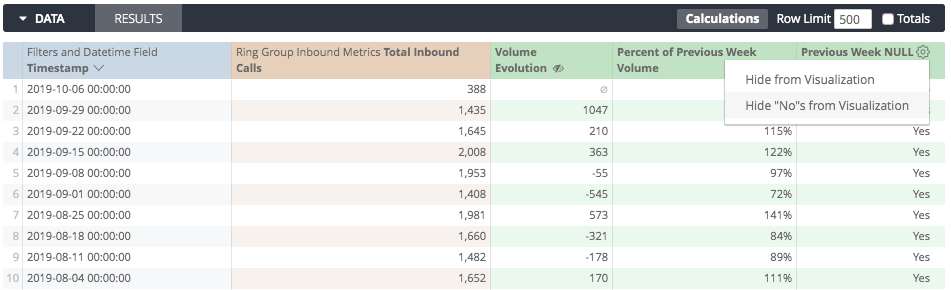## Considerations when using Custom Table Calculations

• All the fields you use in your custom table calculations MUST be a part of your initial query.
• Formulas must be in lowercase. ROUND will not work, but round will.
• Custom table calculations will only operate over rows that are returned in your query. If there is a 500-row limit, the 501st row will not be considered.
• If you add a totals row to your data table, some custom table calculations that perform aggregations, such as calculations using percentile or median, might not add up as you expect. This is because custom table calculations calculate totals using the values in the total row, not using the values in the data column.
• Always use leading zeros for decimals less than 1. For example, 0.95 will work, but .95 will cause an error.
• Using the Command-K or Ctrl+K shortcut will clear all custom table calculations, including custom fields. To reinstate your custom table calculations, hit the “back” button on your browser. You may also need to re-run your query.